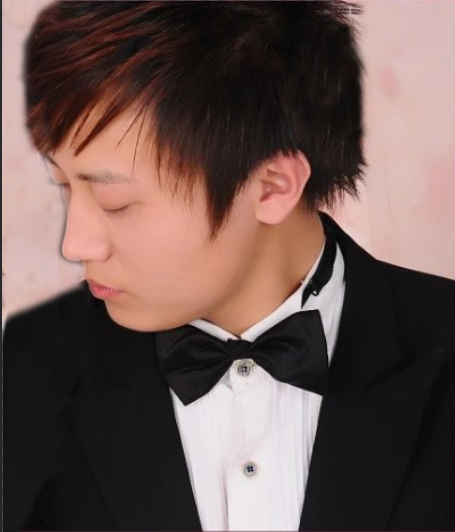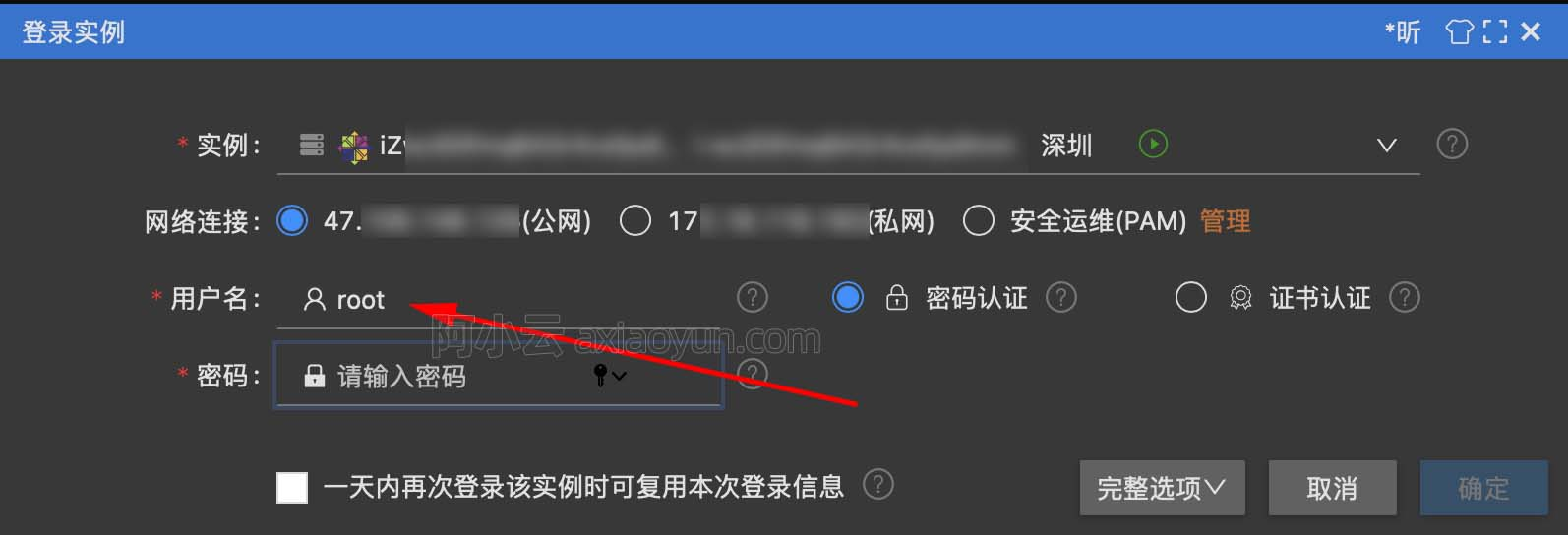+关注继续查看

1. RNN概述

在前面讲到的DNN和CNN中，训练样本的输入和输出是比较的确定的。但是有一类问题DNN和CNN不好解决，就是训练样本输入是连续的序列,且序列的长短不一，比如基于时间的序列：一段段连续的语音，一段段连续的手写文字。这些序列比较长，且长度不一，比较难直接的拆分成一个个独立的样本来通过DNN/CNN进行训练。

而对于这类问题，RNN则比较的擅长。那么RNN是怎么做到的呢？RNN假设我们的样本是基于序列的。比如是从序列索引1到序列索引ττ的。对于这其中的任意序列索引号tt,它对应的输入是对应的样本序列中的x(t)x(t)。而模型在序列索引号tt位置的隐藏状态h(t)h(t)，则由x(t)x(t)和在t1t−1位置的隐藏状态h(t1)h(t−1)共同决定。在任意序列索引号tt，我们也有对应的模型预测输出o(t)o(t)。通过预测输出o(t)o(t)和训练序列真实输出y(t)y(t),以及损失函数L(t)L(t)，我们就可以用DNN类似的方法来训练模型，接着用来预测测试序列中的一些位置的输出。

下面我们来看看RNN的模型。

2. RNN模型

RNN模型有比较多的变种，这里介绍最主流的RNN模型结构如下：上图中左边是RNN模型没有按时间展开的图，如果按时间序列展开，则是上图中的右边部分。我们重点观察右边部分的图。

这幅图描述了在序列索引号tt附近RNN的模型。其中：

1）x(t)x(t)代表在序列索引号tt时训练样本的输入。同样的，x(t1)x(t−1)x(t+1)x(t+1)代表在序列索引号t1t−1t+1t+1时训练样本的输入。

2）h(t)h(t)代表在序列索引号tt时模型的隐藏状态。h(t)h(t)x(t)x(t)h(t1)h(t−1)共同决定。

3）o(t)o(t)代表在序列索引号tt时模型的输出。o(t)o(t)只由模型当前的隐藏状态h(t)h(t)决定。

4）L(t)L(t)代表在序列索引号tt时模型的损失函数。

5）y(t)y(t)代表在序列索引号tt时训练样本序列的真实输出。

6）U,W,VU,W,V这三个矩阵是我们的模型的线性关系参数，它在整个RNN网络中是共享的，这点和DNN很不相同。 也正因为是共享了，它体现了RNN的模型的“循环反馈”的思想。

3. RNN前向传播算法

有了上面的模型，RNN的前向传播算法就很容易得到了。

对于任意一个序列索引号tt，我们隐藏状态h(t)h(t)x(t)x(t)h(t1)h(t−1)得到：

h(t)=σ(z(t))=σ(Ux(t)+Wh(t1)+b)h(t)=σ(z(t))=σ(Ux(t)+Wh(t−1)+b)

其中σσ为RNN的激活函数，一般为tanhtanhbb为线性关系的偏倚。

序列索引号tt时模型的输出o(t)o(t)的表达式比较简单：

o(t)=Vh(t)+co(t)=Vh(t)+c

在最终在序列索引号tt时我们的预测输出为:

y^(t)=σ(o(t))y^(t)=σ(o(t))

通常由于RNN是识别类的分类模型，所以上面这个激活函数一般是softmax。

通过损失函数L(t)L(t)，比如对数似然损失函数，我们可以量化模型在当前位置的损失，即y^(t)y^(t)y(t)y(t)的差距。

4. RNN反向传播算法推导

有了RNN前向传播算法的基础，就容易推导出RNN反向传播算法的流程了。RNN反向传播算法的思路和DNN是一样的，即通过梯度下降法一轮轮的迭代，得到合适的RNN模型参数U,W,V,b,cU,W,V,b,c。由于我们是基于时间反向传播，所以RNN的反向传播有时也叫做BPTT(back-propagation through time)。当然这里的BPTT和DNN也有很大的不同点，即这里所有的U,W,V,b,cU,W,V,b,c在序列的各个位置是共享的，反向传播时我们更新的是相同的参数。

为了简化描述，这里的损失函数我们为对数损失函数，输出的激活函数为softmax函数，隐藏层的激活函数为tanh函数。

对于RNN，由于我们在序列的每个位置都有损失函数，因此最终的损失LL为：

L=t=1τL(t)L=∑t=1τL(t)

其中V,c,V,c,的梯度计算是比较简单的：

Lc=t=1τL(t)c=t=1τL(t)o(t)o(t)c=t=1τy^(t)y(t)∂L∂c=∑t=1τ∂L(t)∂c=∑t=1τ∂L(t)∂o(t)∂o(t)∂c=∑t=1τy^(t)−y(t)
LV=t=1τL(t)V=t=1τL(t)o(t)o(t)V=t=1τ(y^(t)y(t))(h(t))T∂L∂V=∑t=1τ∂L(t)∂V=∑t=1τ∂L(t)∂o(t)∂o(t)∂V=∑t=1τ(y^(t)−y(t))(h(t))T

但是W,U,bW,U,b的梯度计算就比较的复杂了。从RNN的模型可以看出，在反向传播时，在在某一序列位置t的梯度损失由当前位置的输出对应的梯度损失和序列索引位置t+1t+1时的梯度损失两部分共同决定。对于WW在某一序列位置t的梯度损失需要反向传播一步步的计算。我们定义序列索引tt位置的隐藏状态的梯度为：

δ(t)=Lh(t)δ(t)=∂L∂h(t)

这样我们可以像DNN一样从δ(t+1)δ(t+1)递推δ(t)δ(t) 。

δ(t)=Lo(t)o(t)h(t)+Lh(t+1)h(t+1)h(t)=VT(y^(t)y(t))+WTδ(t+1)diag(1(h(t+1))2)δ(t)=∂L∂o(t)∂o(t)∂h(t)+∂L∂h(t+1)∂h(t+1)∂h(t)=VT(y^(t)−y(t))+WTδ(t+1)diag(1−(h(t+1))2)

对于δ(τ)δ(τ)，由于它的后面没有其他的序列索引了，因此有：

δ(τ)=Lo(τ)o(τ)h(τ)=VT(y^(τ)y(τ))δ(τ)=∂L∂o(τ)∂o(τ)∂h(τ)=VT(y^(τ)−y(τ))

有了δ(t)δ(t),计算W,U,bW,U,b就容易了，这里给出W,U,bW,U,b的梯度计算表达式：

LW=t=1τLh(t)h(t)W=t=1τdiag(1(h(t))2)δ(t)(h(t1))T∂L∂W=∑t=1τ∂L∂h(t)∂h(t)∂W=∑t=1τdiag(1−(h(t))2)δ(t)(h(t−1))T
Lb=t=1τLh(t)h(t)b=t=1τdiag(1(h(t))2)δ(t)∂L∂b=∑t=1τ∂L∂h(t)∂h(t)∂b=∑t=1τdiag(1−(h(t))2)δ(t)
LU=t=1τLh(t)h(t)U=t=1τdiag(1(h(t))2)δ(t)(x(t))T∂L∂U=∑t=1τ∂L∂h(t)∂h(t)∂U=∑t=1τdiag(1−(h(t))2)δ(t)(x(t))T

除了梯度表达式不同，RNN的反向传播算法和DNN区别不大，因此这里就不再重复总结了。

5. RNN小结

上面总结了通用的RNN模型和前向反向传播算法。当然，有些RNN模型会有些不同，自然前向反向传播的公式会有些不一样，但是原理基本类似。

RNN虽然理论上可以很漂亮的解决序列数据的训练，但是它也像DNN一样有梯度消失时的问题，当序列很长的时候问题尤其严重。因此，上面的RNN模型一般不能直接用于应用领域。在语音识别，手写书别以及机器翻译等NLP领域实际应用比较广泛的是基于RNN模型的一个特例LSTM，下一篇我们就来讨论LSTM模型。9497 06336 014377 0windows server 2008阿里云ECS服务器安全设置

9054 013186 07386 054292 06894 021908 04012 0
3576

0

《2021云上架构与运维峰会演讲合集》

《零基础CSS入门教程》

《零基础HTML入门教程》